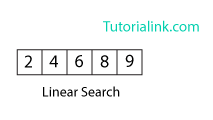DS

# Algorithm for Searching an element into an array

An algorithm is a step-by-step procedure or method for solving a problem by a computer in a given number of steps. The steps of an algorithm may include repetition depending upon the problem for which the algorithm is being developed. The algorithm is written in human readable and understandable form. To search an element in a given array, it can be done in two ways Linear search and Binary search.

## Linear Search

A linear search is the basic and simple search algorithm.
A linear search searches an element or value from an array till the desired element or value is not found and it searches in a sequence order.
It compares the element with all the other elements given in the list and if the element is matched it returns the value index else it return -1.
Linear Search is applied on the unsorted or unordered list when there are fewer elements in a list.

### Example

To search the element 9 it will go step by step in a sequence order.``````function findIndex(values, target)
{
for(var i = 0; i < values.length; ++i)
{
if (values[i] == target)
{
return i;
}
}
return -1;
}
//call the function findIndex with array and number to be searched
findIndex([ 2 , 4 , 6 , 8 , 9 ] , 9) ;``````

## Binary Search

Binary Search is applied on the sorted array or list.
In binary search, we first compare the value with the elements in the middle position of the array.
If the value is matched, then we return the value. If the value is less than the middle element, then it must lie in the lower half of the array and if it's greater than the element then it must lie in the upper half of the array.
We repeat this procedure on the lower (or upper) half of the array.
Binary Search is useful when there are large numbers of elements in an sorted array.

### Example

To search an element 9 from the sorted array or list.``````function findIndex(values, target)
{
return binarySearch(values, target, 0, values.length - 1);
};

function binarySearch(values, target, start, end)
{
if (start > end) { return -1; } //does not exist

var middle = Math.floor((start + end) / 2);
var value = values[middle];

if (value > target) { return binarySearch(values, target, start, middle-1); }
if (value < target) { return binarySearch(values, target, middle+1, end); }
return middle; //found!
}

findIndex([2, 4, 6, 8, 9, 10], 9);``````

In the above program logic, we are first comparing the middle number of the list, with the target, if it matches we return. If it doesn't, we see whether the middle number is greater than or smaller than the target.

If the Middle number is greater than the Target, we start the binary search again, but this time on the left half of the list, that is from the start of the list to the middle, not beyond that.

If the Middle number is smaller than the Target, we start the binary search again, but on the right half of the list, that is from the middle of the list to the end of the list.